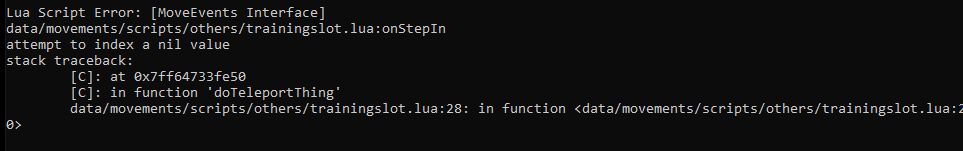# TFS 1.X+Training slot script error

#### ralke

##### (҂ ͠❛ ෴ ͡❛)ᕤ
Hi! I got this scripts to automatically teleport players to training zones, but I encounter this error. Not sure, but I think that it's triggered when the training rooms are full.Lua:
``````local config = {
{x=168,y=472,z=8},
{x=187,y=472,z=8},
{x=206,y=472,z=8},
{x=225,y=472,z=8},
{x=168,y=490,z=8},
{x=187,y=490,z=8},
{x=206,y=490,z=8},
{x=225,y=490,z=8},
{x=168,y=508,z=8},
{x=187,y=508,z=8},
{x=206,y=508,z=8},
{x=225,y=508,z=8},
{x=168,y=526,z=8},
{x=187,y=526,z=8},
{x=206,y=526,z=8},
{x=225,y=526,z=8}
}

function onStepIn(cid, item, position, lastPosition, fromPosition, toPosition)
for _, pos in pairs(config) do
if not isPlayer(getTopCreature(pos).uid) then
if doTeleportThing(cid, pos) then
return true
end
end
end
doTeleportThing(cid, fromPosition)
doSendMagicEffect(getThingPos(cid), CONST_ME_TELEPORT)
doCreatureSay(cid, "The training area is full.", TALKTYPE_MONSTER, nil, nil, fromPosition)
return true
end``````

How can I possibly fix this to don't make this error appear again? Or optimize this script a little bit, it will use the same coordinates.

Solution
change
Lua:
``function onStepIn(cid, item, position, lastPosition, fromPosition, toPosition)``
to
Lua:
``function onStepIn(cid, item, position, fromPosition)``

I'm 99% sure those other position parameters don't exist, and thus you're getting a nil value
change
Lua:
``function onStepIn(cid, item, position, lastPosition, fromPosition, toPosition)``
to
Lua:
``function onStepIn(cid, item, position, fromPosition)``

I'm 99% sure those other position parameters don't exist, and thus you're getting a nil value

•Sarah Wesker
change
Lua:
``function onStepIn(cid, item, position, lastPosition, fromPosition, toPosition)``
to
Lua:
``function onStepIn(cid, item, position, fromPosition)``

I'm 99% sure those other position parameters don't exist, and thus you're getting a nil value

Thanks you @Xikini I will do the proper testings asap, for the moment, I think you're right. If I found something else weird I will let you know.
Regards!Post automatically merged:

Edit: Work as intended, solved!

Last edited:
•Xikini